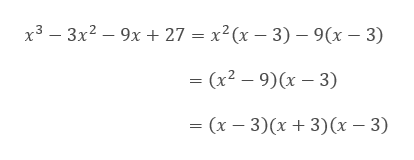# f(x)=x3-3x2-9x+27 determine the zeros, their multiplicity, and whether the graph will touch or cross the x-axis at each zero. Then, determine the end behavior of the function and sketch a graph of the function.

Question

f(x)=x3-3x2-9x+27 determine the zeros, their multiplicity, and whether the graph will touch or cross the x-axis at each zero. Then, determine the end behavior of the function and sketch a graph of the function.

check_circleExpert Solution
Step 1

Given function:

Step 2

To Find: Zeros of the function, their multiplicity and the graph.

Now, we factorise to get the zeros.help_outlineImage Transcriptioncloseх3 — 3x2 — 9х + 27 %3D х?(х — 3) — 9(х — 3) (x2 — 9)(х — 3) — (х — 3)(х + 3)(х — 3) fullscreen
Step 3

Thus, the zeros of the given ...

### Want to see the full answer?

See Solution

#### Want to see this answer and more?

Solutions are written by subject experts who are available 24/7. Questions are typically answered within 1 hour*

See Solution
*Response times may vary by subject and question
Tagged in

### Geometry